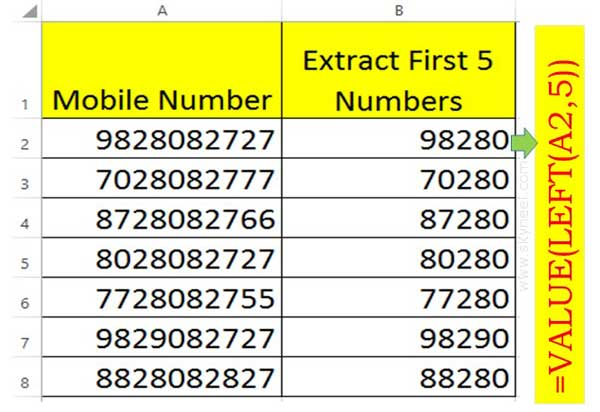# How to Extract few Numbers using Excel Functions

Excel allows you to insert different types of data format in cell of active worksheet. You can extract few numbers using Excel Functions.

Excel is a great application spreadsheet software which is used for complex calculations. During working on the Excel you can feed different kind of data. If you have any number is range of cells and want to extract few numbers using Excel functions. In this article we have to guide how to extract few numbers with the help of Value and Left functions in Excel.

All we know function are very useful during doing complex calculation or getting the required result. If you have good command on Excel then you have to easily prepare different kind of formats which have lot of functions. All we know that you can easily enter different kind of data types in the cell. You can easily format the cells, applying conditional formatting, apply restriction on selected cell contents, apply lot of functions as per your need. All these commands and function are useful to get the required result.

Must Read: How to use PMT Function in Excel

## How to Extract few Numbers using Excel Functions

If you have good command on Excel commands and function then you will be able to easily resolve lot of complex problem which is related to calculation, data analysis and many more. For. eg: If you have range of list in which different mobile numbers are entered. Now you want to extract first five numbers and also want to keep same value format on resulted cells. In that situation most of the person have to simply use Left function to get the result but format of the resulted values in changed. You will get result in the text format at the place of number.

Now, a question arise in your mind how to extract few numbers using Excel functions during working on the sheet. There is no need to worry you just have to take few given simple steps which helps you to easily extract few numbers using Excel functions and result format does not change.Step 1: Create a new worksheet to start the process how to extract few numbers using Excel functions.

Step 2: Take cursor in cell B2 and type the following formula to extract first five numbers of given mobile number.

=VALUE(LEFT(A2,5))

Step 3: After typing the above given formula press enter to get the result. Now, drag the resulted cell formula for rest of cells to get the result of all cells.

I hope after reading this guide you can easily extract few numbers using Excel formulas. You can use Value and Left function to get the accurate result as per the given above situation. If you have any suggestion regarding this guide then please write us in the comment box. Thanks to all.